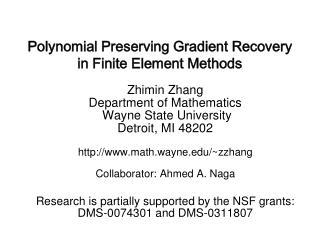DownloadDownload PresentationPolynomial Preserving Gradient Recovery in Finite Element Methods

Polynomial Preserving Gradient Recovery in Finite Element Methods

Télécharger la présentationPolynomial Preserving Gradient Recovery in Finite Element Methods

- - - - - - - - - - - - - - - - - - - - - - - - - - - E N D - - - - - - - - - - - - - - - - - - - - - - - - - - -
Presentation Transcript

1. Polynomial Preserving Gradient Recovery in Finite Element Methods Zhimin Zhang Department of Mathematics Wayne State University Detroit, MI 48202 http://www.math.wayne.edu/~zzhang Collaborator: Ahmed A. Naga Research is partially supported by the NSF grants: DMS-0074301 and DMS-0311807

2. Polynomial Preserving Recovery Motivation The ZZ patch recovery is not perfect! 1. Difficulty on the boundary, especially curved boundary. 2. Not polynomial preserving. 3. Superconvergence cannot be guaranteed in general. EVERY AVERAGING WORKS! C. Carstensen, 2002

3. Polynomial Preserving Recovery Recovery operator Gh: Sh,k Sh,k× Sh,k. Nodal values of Ghuh are defined by 1) At a vertex: pk+1(0, 0; zi); 2) At an edge node between two vertices zi1 andzi2: pk+1(x1, y1; zi1) + (1-)pk+1(x2, y2; zi2), 0<<1; 3)At an interior node on the triangle formed byzij's: Here pk+1(.; zi) is the polynomial from a least-squares fitting of uh at some nodal points surroundingzi . Ghuh is defined on the whole domain by interpolation using the original basis functions of Sh,k . The Procedure

4. Linear Element

6. Cubic Element

7. Q1 Element

8. Q2 and Q2’ Element

9. p27

10. P23a-c Mesh geometry(a-c)

11. p23d-e Mesh geometry(d-e)

12. p23f-g Mesh geometry(f-g)

13. Polynomial Preserving Recovery Examples on Uniform Mesh I • Vertex valueGhu(zi) for linear element. • I.1. Regular pattern. • I.2. Chevron pattern. • Regular pattern, same as ZZ and simple averaging. • Chevron pattern, all three are different.

14. p18

15. Polynomial Preserving Recovery Examples on Uniform Mesh II • Quadratic element on regular pattern. • II.1. At a vertex; • II.2. At a horizontal edge center; • II.3. At a vertical edge center; • II.4. At a diagonal edge center. • In general, • where zij are nodes involved. • If zij distribute symmetrically around zi, then coefficients • cj(zi) distribute anti-symmetrically.

16. p19

17. p20

18. p21

19. p22

20. Polynomial Preserving Recovery Polynomial preserving Property • i, a union of elements that covers all nodes needed for • the recovery ofGhuh(zi). • Theorem 1.Letu Wk+2 (i), then • If zi is a grid symmetry point and u Wk+2 (i) with k=2r, • then • The ZZ patch recovery does not have this property.

21. Polynomial Preserving Recovery Key Observation • Ghu(z): difference quotient on translation invariant mesh, • Example: Linear element, regular pattern, vertex O: • Translations are in the directions of

22. Polynomial Preserving Recovery • Theorem 2.Let the finite element space Sh,k be transla- • tion invariant in directions required by the recovery opera- • tor Gh on D, let u Wk+2 (), and let A(u-uh,v)=0 • for vS0h,k(). Assume that Theorem 5.5.2 in Wahlbin's • book is applicable. Then on any interior region 0, • there is a constant C independent of h and u such that for • some s 0 and q1, Superconvergence Property I

23. Polynomial Preserving Recovery • Th: triangulation for. • Condition (): Th = T1,h  T2,h with • every two adjacent triangles inside T1,h form an O(h1+) • (>0) parallelogram; • 2) |2,h| = O(h),  > 0; 2,h = T2,h. • Observation: Usually, a mesh produced by an automatic mesh generator satisfies Condition (). Irregular Grids

24. Polynomial Preserving Recovery • Theorem 3.Let u W3() be the solution of • A(u, v) = (f, v), v  H1(), • let uhSh,1 be the finite element approximation, and let • Th satisfies Condition(). Assume that f and all coeffi- • cients of the operator A are smooth. Then Superconvergence Property II

25. Polynomial Preserving Recovery Comparison with ZZ • Linear element on Chevron pattern: • O(h2) compare withO(h) for ZZ. • 2. Quadratic element on regular patter at edge centers: • O(h4) compare withO(h2) for ZZ. • 3. Mesh distortion at a vertex for ZZ:

26. P24_1 Mesh distortion

27. Polynomial Preserving Recovery Numerical Tests • Case 1.The Poisson equation with zero boundary condi- • tion on the unit square with the exact solution • u(x, y) = x (1 - x) y (1 - y). • Case 2.The exact solution isu(x, y) = sinx siny. • -  u = 22 sinxsinyin  = [0, 1]2, u = 0 on .

28. p24_2 Linear element (Chevron) case 1

29. p24_3 Linear element (Chevron) case 2

30. p25_1 Quadratic element case 1

31. p25_2 Quadratic element case 2

32. Polynomial Preserving Recovery ZZ Patch Recovery in Industry • Purpose: smoothing and adaptive remeshing. • ANSYS • MCS/NASTRAN-Marc • Pro/MECHANICA (product of Parametric Technology) • I-DEAS (product of SDRC, part of EDS) • COMET-AR(NASA):COmputational MEchanics Testbed With Adaptive Refinement